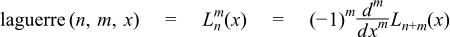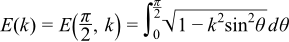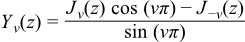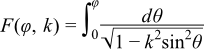Boost C++ Libraries

...one of the most highly regarded and expertly designed C++ library projects in the world.

This is the documentation for an old version of boost. Click here for the latest Boost documentation.

TR1 C Functions Quick Reference

Supported TR1 Functions
namespace boost{ namespace math{ namespace tr1{ extern "C"{

// [5.2.1.1] associated Laguerre polynomials:
double assoc_laguerre(unsigned n, unsigned m, double x);
float assoc_laguerref(unsigned n, unsigned m, float x);
long double assoc_laguerrel(unsigned n, unsigned m, long double x);

// [5.2.1.2] associated Legendre functions:
double assoc_legendre(unsigned l, unsigned m, double x);
float assoc_legendref(unsigned l, unsigned m, float x);
long double assoc_legendrel(unsigned l, unsigned m, long double x);

// [5.2.1.3] beta function:
double beta(double x, double y);
float betaf(float x, float y);
long double betal(long double x, long double y);

// [5.2.1.4] (complete) elliptic integral of the first kind:
double comp_ellint_1(double k);
float comp_ellint_1f(float k);
long double comp_ellint_1l(long double k);

// [5.2.1.5] (complete) elliptic integral of the second kind:
double comp_ellint_2(double k);
float comp_ellint_2f(float k);
long double comp_ellint_2l(long double k);

// [5.2.1.6] (complete) elliptic integral of the third kind:
double comp_ellint_3(double k, double nu);
float comp_ellint_3f(float k, float nu);
long double comp_ellint_3l(long double k, long double nu);

// [5.2.1.8] regular modified cylindrical Bessel functions:
double cyl_bessel_i(double nu, double x);
float cyl_bessel_if(float nu, float x);
long double cyl_bessel_il(long double nu, long double x);

// [5.2.1.9] cylindrical Bessel functions (of the first kind):
double cyl_bessel_j(double nu, double x);
float cyl_bessel_jf(float nu, float x);
long double cyl_bessel_jl(long double nu, long double x);

// [5.2.1.10] irregular modified cylindrical Bessel functions:
double cyl_bessel_k(double nu, double x);
float cyl_bessel_kf(float nu, float x);
long double cyl_bessel_kl(long double nu, long double x);

// [5.2.1.11] cylindrical Neumann functions;
// cylindrical Bessel functions (of the second kind):
double cyl_neumann(double nu, double x);
float cyl_neumannf(float nu, float x);
long double cyl_neumannl(long double nu, long double x);

// [5.2.1.12] (incomplete) elliptic integral of the first kind:
double ellint_1(double k, double phi);
float ellint_1f(float k, float phi);
long double ellint_1l(long double k, long double phi);

// [5.2.1.13] (incomplete) elliptic integral of the second kind:
double ellint_2(double k, double phi);
float ellint_2f(float k, float phi);
long double ellint_2l(long double k, long double phi);

// [5.2.1.14] (incomplete) elliptic integral of the third kind:
double ellint_3(double k, double nu, double phi);
float ellint_3f(float k, float nu, float phi);
long double ellint_3l(long double k, long double nu, long double phi);

// [5.2.1.15] exponential integral:
double expint(double x);
float expintf(float x);
long double expintl(long double x);

// [5.2.1.16] Hermite polynomials:
double hermite(unsigned n, double x);
float hermitef(unsigned n, float x);
long double hermitel(unsigned n, long double x);

// [5.2.1.18] Laguerre polynomials:
double laguerre(unsigned n, double x);
float laguerref(unsigned n, float x);
long double laguerrel(unsigned n, long double x);

// [5.2.1.19] Legendre polynomials:
double legendre(unsigned l, double x);
float legendref(unsigned l, float x);
long double legendrel(unsigned l, long double x);

// [5.2.1.20] Riemann zeta function:
double riemann_zeta(double);
float riemann_zetaf(float);
long double riemann_zetal(long double);

// [5.2.1.21] spherical Bessel functions (of the first kind):
double sph_bessel(unsigned n, double x);
float sph_besself(unsigned n, float x);
long double sph_bessell(unsigned n, long double x);

// [5.2.1.22] spherical associated Legendre functions:
double sph_legendre(unsigned l, unsigned m, double theta);
float sph_legendref(unsigned l, unsigned m, float theta);
long double sph_legendrel(unsigned l, unsigned m, long double theta);

// [5.2.1.23] spherical Neumann functions;
// spherical Bessel functions (of the second kind):
double sph_neumann(unsigned n, double x);
float sph_neumannf(unsigned n, float x);
long double sph_neumannl(unsigned n, long double x);

}}}} // namespaces

In addition sufficient additional overloads of the double versions of the above functions are provided, so that calling the function with any mixture of float, double, long double, or integer arguments is supported, with the return type determined by the result type calculation rules.

For example:

expintf(2.0f);  // float version, returns float.
expint(2.0f);   // also calls the float version and returns float.
expint(2.0);    // double version, returns double.
expintl(2.0L);  // long double version, returns a long double.
expint(2.0L);   // also calls the long double version.
expint(2);      // integer argument is treated as a double, returns double.
Quick Reference
// [5.2.1.1] associated Laguerre polynomials:
double assoc_laguerre(unsigned n, unsigned m, double x);
float assoc_laguerref(unsigned n, unsigned m, float x);
long double assoc_laguerrel(unsigned n, unsigned m, long double x);

The assoc_laguerre functions return:// [5.2.1.2] associated Legendre functions:
double assoc_legendre(unsigned l, unsigned m, double x);
float assoc_legendref(unsigned l, unsigned m, float x);
long double assoc_legendrel(unsigned l, unsigned m, long double x);

The assoc_legendre functions return:// [5.2.1.3] beta function:
double beta(double x, double y);
float betaf(float x, float y);
long double betal(long double x, long double y);

Returns the beta function of x and y:// [5.2.1.4] (complete) elliptic integral of the first kind:
double comp_ellint_1(double k);
float comp_ellint_1f(float k);
long double comp_ellint_1l(long double k);

Returns the complete elliptic integral of the first kind of k:// [5.2.1.5] (complete) elliptic integral of the second kind:
double comp_ellint_2(double k);
float comp_ellint_2f(float k);
long double comp_ellint_2l(long double k);

Returns the complete elliptic integral of the second kind of k:// [5.2.1.6] (complete) elliptic integral of the third kind:
double comp_ellint_3(double k, double nu);
float comp_ellint_3f(float k, float nu);
long double comp_ellint_3l(long double k, long double nu);

Returns the complete elliptic integral of the third kind of k and nu:// [5.2.1.8] regular modified cylindrical Bessel functions:
double cyl_bessel_i(double nu, double x);
float cyl_bessel_if(float nu, float x);
long double cyl_bessel_il(long double nu, long double x);

Returns the modified bessel function of the first kind of nu and x:// [5.2.1.9] cylindrical Bessel functions (of the first kind):
double cyl_bessel_j(double nu, double x);
float cyl_bessel_jf(float nu, float x);
long double cyl_bessel_jl(long double nu, long double x);

Returns the bessel function of the first kind of nu and x:// [5.2.1.10] irregular modified cylindrical Bessel functions:
double cyl_bessel_k(double nu, double x);
float cyl_bessel_kf(float nu, float x);
long double cyl_bessel_kl(long double nu, long double x);

Returns the modified bessel function of the second kind of nu and x:// [5.2.1.11] cylindrical Neumann functions;
// cylindrical Bessel functions (of the second kind):
double cyl_neumann(double nu, double x);
float cyl_neumannf(float nu, float x);
long double cyl_neumannl(long double nu, long double x);

Returns the bessel function of the second kind (Neumann function) of nu and x:// [5.2.1.12] (incomplete) elliptic integral of the first kind:
double ellint_1(double k, double phi);
float ellint_1f(float k, float phi);
long double ellint_1l(long double k, long double phi);

Returns the incomplete elliptic integral of the first kind of k and phi:// [5.2.1.13] (incomplete) elliptic integral of the second kind:
double ellint_2(double k, double phi);
float ellint_2f(float k, float phi);
long double ellint_2l(long double k, long double phi);

Returns the incomplete elliptic integral of the second kind of k and phi:// [5.2.1.14] (incomplete) elliptic integral of the third kind:
double ellint_3(double k, double nu, double phi);
float ellint_3f(float k, float nu, float phi);
long double ellint_3l(long double k, long double nu, long double phi);

Returns the incomplete elliptic integral of the third kind of k, nu and phi:// [5.2.1.15] exponential integral:
double expint(double x);
float expintf(float x);
long double expintl(long double x);

Returns the exponential integral Ei of x:// [5.2.1.16] Hermite polynomials:
double hermite(unsigned n, double x);
float hermitef(unsigned n, float x);
long double hermitel(unsigned n, long double x);

Returns the n'th Hermite polynomial of x:// [5.2.1.18] Laguerre polynomials:
double laguerre(unsigned n, double x);
float laguerref(unsigned n, float x);
long double laguerrel(unsigned n, long double x);

Returns the n'th Laguerre polynomial of x:// [5.2.1.19] Legendre polynomials:
double legendre(unsigned l, double x);
float legendref(unsigned l, float x);
long double legendrel(unsigned l, long double x);

Returns the l'th Legendre polynomial of x:// [5.2.1.20] Riemann zeta function:
double riemann_zeta(double);
float riemann_zetaf(float);
long double riemann_zetal(long double);

Returns the Riemann Zeta function of x:// [5.2.1.21] spherical Bessel functions (of the first kind):
double sph_bessel(unsigned n, double x);
float sph_besself(unsigned n, float x);
long double sph_bessell(unsigned n, long double x);

Returns the spherical Bessel function of the first kind of x jn(x):// [5.2.1.22] spherical associated Legendre functions:
double sph_legendre(unsigned l, unsigned m, double theta);
float sph_legendref(unsigned l, unsigned m, float theta);
long double sph_legendrel(unsigned l, unsigned m, long double theta);

Returns the spherical associated Legendre function of l, m and theta:// [5.2.1.23] spherical Neumann functions;
// spherical Bessel functions (of the second kind):
double sph_neumann(unsigned n, double x);
float sph_neumannf(unsigned n, float x);
long double sph_neumannl(unsigned n, long double x);

Returns the spherical Neumann function of x yn(x):Currently Unsupported TR1 Functions
// [5.2.1.7] confluent hypergeometric functions:
double conf_hyperg(double a, double c, double x);
float conf_hypergf(float a, float c, float x);
long double conf_hypergl(long double a, long double c, long double x);

// [5.2.1.17] hypergeometric functions:
double hyperg(double a, double b, double c, double x);
float hypergf(float a, float b, float c, float x);
long double hypergl(long double a, long double b, long double c,
long double x);
NoteThese two functions are not implemented as they are not believed to be numerically stable.